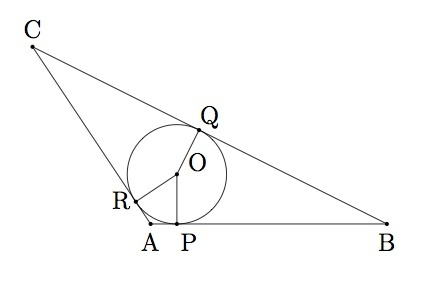# Inscribing a circle in a triangle II

Alignments to Content Standards: G-C.A.3

Suppose a circle with center $O$ is inscribed inside triangle $ABC$ as in the picture below:Also pictured above are the three radii of the inscribed circle which meet the triangle at the three points $P$, $Q$, and $R$.

1. Show that triangle $BPO$ is congruent to triangle $BQO$.
2. Show that ray $\overrightarrow{BO}$ is the bisector of angle $B$.
3. Show that rays $\overrightarrow{AO}$and $\overrightarrow{CO}$ bisect the respective angles $A$ and $C$.
4. Show that if a circle is inscribed in a triangle then the center of that circle is contained on all three angle bisectors for the angles of the triangle.

## IM Commentary

This task focuses on a remarkable fact which comes out of the construction of the inscribed circle in a triangle: the angle bisectors of the three angles of triangle $ABC$ all meet in a point. Students will need to use the fact that the radii of the inscribed circle at points $P$, $Q$, and $R$ are perpendicular to lines $\overleftrightarrow{AB}$, $\overleftrightarrow{BC}$, and $\overleftrightarrow{AC}$ respectively.

This task is intended mostly for instruction purposes because it relies on previous work with rigid motions of the plane and also uses the fact that the radius of a circle at a point $D$ meets the tangent line to the circle at $D$ perpendicularly. This task could be rewarding for students even if only approached from a construction point of view. Finding the center of the inscribed circle and seeing the three angle bisectors meeting in a point are both valuable learning experiences which might stimulate further thought. It might also be helpful to go through the steps of the problem with an equilateral triangle or a right isosceles triangle- the added symmetry of these examples would help visualize the situation and then afterward the scalene case might be easier to approach.

## Solution

1. Note first that the lines containing each side of triangle $ABC$ meet the circle in a single point and so they are tangent lines to the circle. So $\overline{OQ}$ is perpendicular to $\overleftrightarrow{BC}$ and $\overline{OP}$ is perpendicular to $\overleftrightarrow{AB}$ Hence angles $BPO$ and $BQO$ are congruent and they are both right angles. We also know that $\overline{OP}$ is congruent to $\overline{OQ}$ because both are radii of the same circle. Finally, $\overline{OB}$ is common to triangles $BPO$ and $BQO$. Since triangles $BQO$ and $BPO$ are right triangles whose hypotenuses are congruent and they share one congruent leg, the Pythagorean theorem implies that the remaining pair of legs, $\overline{PB}$ and $\overline{QB}$, are also congruent. By SSS we can conclude that triangles $BQO$ and $BPO$ are congruent.

Alternatively, students who know the Hypotenuse-Leg criterion for congruence can observe that triangles $BQO$ and $BPO$ are right triangles which share the same hypotenuse. Legs $\overline{QO}$ and $\overline{PO}$ are congruent and so the Hypotenuse-Leg criterion applies to show that triangles $BQO$ and $BPO$ are congruent.

2. Since triangles $BPO$ and $BQO$ are congruent and angles $QBO$ and $PBO$ are corresponding angles of these triangles they are congruent. This means that $\overrightarrow{BO}$ bisects angle $B$.

3. Triangles $CQO$ and $CRO$ are congruent by the same SSS argument used to show that triangles $BPO$ and $BQO$ are congruent:

1. Side $\overline{OQ}$ is congruent to side $\overline{OR}$ since both are radii of the same circle,
2. Side $\overline{OQ}$ is congruent to itself.
3. Angles $CQO$ and $CRO$ are right angles as shown in part (a) and so the Pythagorean theorem, combined with (i) and (ii) above, implies that the remaining pair of sides, $\overline{CR}$ and $\overline{CQ}$, are congruent.

As in part (a) above, students may also apply the Hypotenuse-Leg criterion to show that triangles $CQO$ and $CRO$ are congruent. These two right triangles share hypotenuse $\overline{CO}$ and have congruent legs $\overline{QO}$ and $\overline{RO}$ since these are both radii of the same circle.

Angles $RCO$ and $QCO$ are corresponding angles of the congruent triangles $RCO$ and $QCO$ so they are congruent. Hence $\overrightarrow{CO}$ bisects angle $C$. The same argument can be repeated a third time to show that $\overrightarrow{AO}$ bisects angle $A$.

4. Parts (b) and (c) show that $\overrightarrow{AO}$ is the bisector of angle $A$, $\overrightarrow{BO}$ is the bisector of angle $B$, and $\overrightarrow{CO}$ is the bisector of angle $C$. These angle bisectors all contain the point $O$ at the center of the inscribed circle. The three concurrent angle bisectors are shown below: